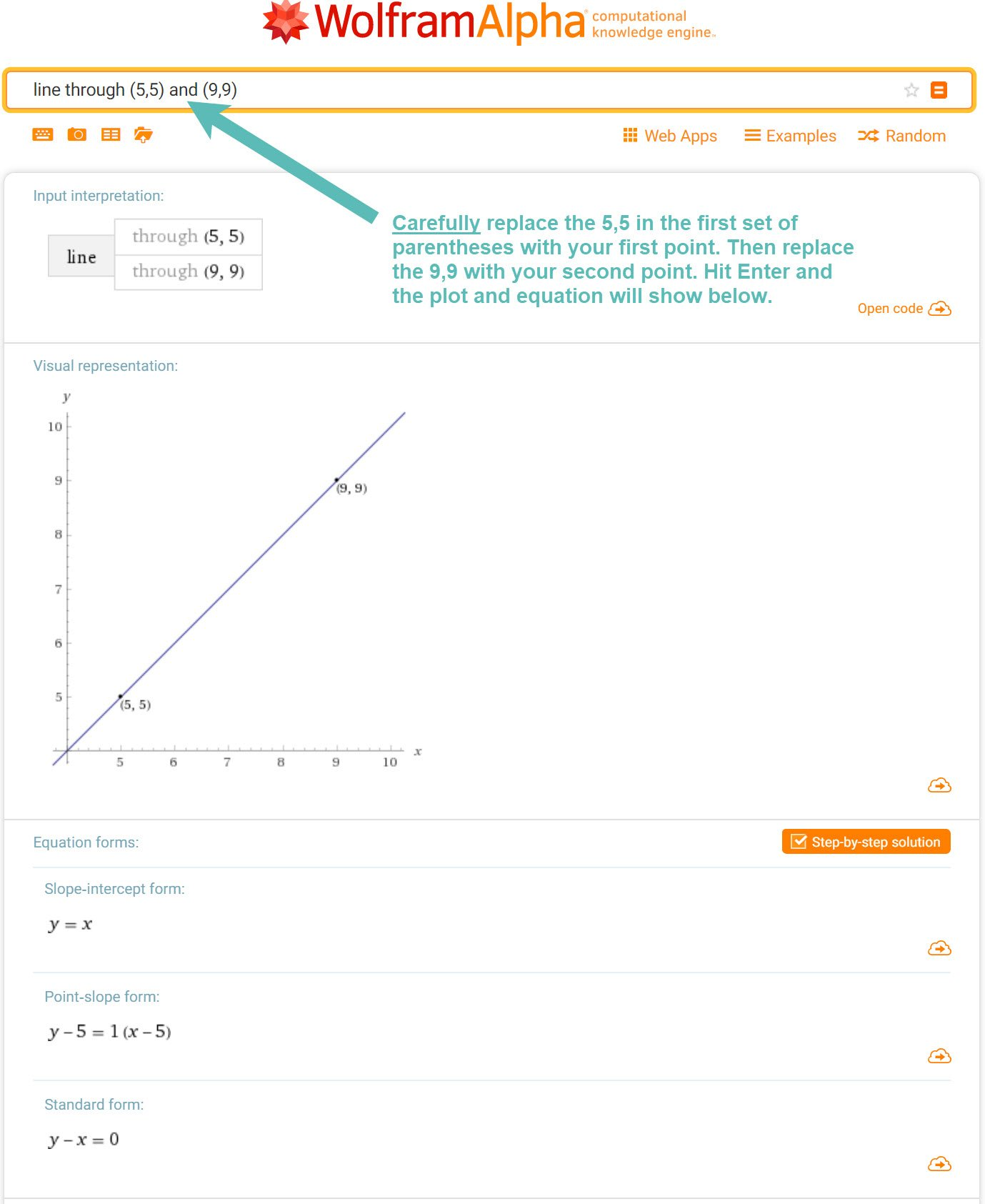Select Page

If you need to find the equation of a line or its plot and you are given just two points, e.g. (5,5)(9,9), the best way to get the right answer is to use the WolframAlpha calculator at this page: https://www.wolframalpha.com/input/?i=line+through+(5,5)+and+(9,9)If you are given the equation of a line and you need to plot it, use this WolframAlpha calculator: https://www.wolframalpha.com/input/?i=line,+slope%3D2.75,+y-intercept%3D.5